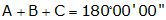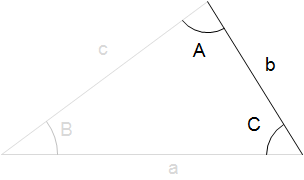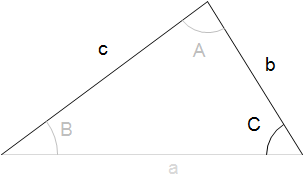## 1. Oblique triangles

### a. Formulae

An oblique triangle is one in which individual angles are greater than 0° and less than 180°. A right triangle is a special oblique triangle in which one angle is exactly 90°.

A plane triangle has six parts: three angles and three sides.Figure B-1 Triangle Parts

Equations for triangle trigonometry are:

 Angle ConditionEquation B-1 Law of SinesEquation B-2 Law of CosinesEquation B-3

A right triangle is a special case where one angle is exactly 90°. If we apply the Law of Cosines, Equation B-3, to a right triangle:The Law of Cosines becomes the Pythagorean Theorem. Figure B-2 Right triangle

### b. Solving triangles

In order to geometrically fix a triangle you must start with at least three parts and one of those must be a side. Why a side? Because fixing the angles alone does not constrain the size of the triangle.These two triangles have identical angles but are different sizes. Figure B-2 Angles Don't Fix Triangle

Starting with three parts of a triangle, there is only one way to begin solving for another of its parts. Once a fourth part is determined, there are more ways to solve the remaing parts.

For example:Given three sides of a triangle, how would you begin solving for other parts of it?Start with the Law of Cosines to solve for one angle. a2=b2+c2-2x(bc)xCos(A) Can then use Law of Cosines or Law of Sines to solve for next angle. Can then use Law of Cosines or Law of Sines to solve for next angle. Last angle can be solved by Law of Cosines, Law of Sines, or Angle ConditionHow about two sides and an included angle?Start with the Law of Cosines to solve for side a (known values in red): a2=b2+c2-2x(bc)xCos(A) Can then use Law of Cosines or Law of Sines to solve for another angle. Last angle can be solved by Law of Cosines, Law of Sines, or Angle Condition

How would you solve these triangles?Two angles and the included side.Two angles an a non-included side.Two sides and a non-included angle.

### c. Area

Another attribute of a triangle is its area which can be determined by one of two equations:

 Using two sides and an included angle.Equation B-4 Using all three sides - Heron's Formula.Equation B-5

Given two parts of a triangle and its area, you should be able to determine its other parts, right?Starting with two sides and an area...Solve angle A using the area equation: Area = 1/2(bc)xCos (A) Then use Law of Cosines to solve side a a2=b2+c2-2(bc)Cos(A) Then.....How about starting with an angle, opposite side, and triangle area?